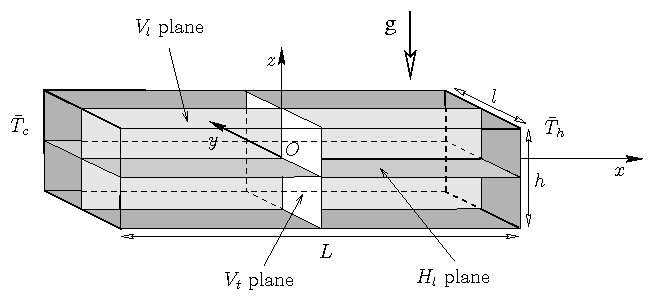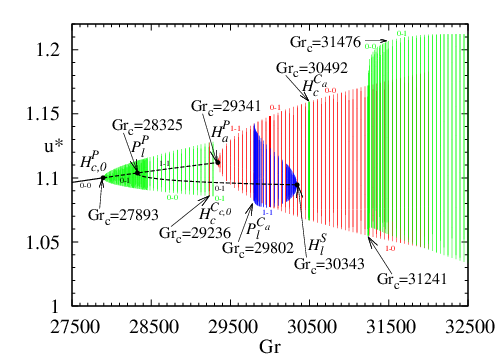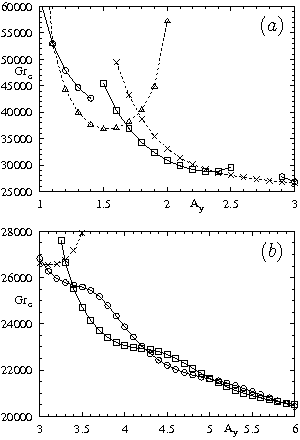# Fluid Mechanics and Acoustics Laboratory - UMR 5509

LMFA - UMR 5509
Laboratoire de Mécanique des Fluides et d’Acoustique
Lyon
France

## Oscillatory instabilities in cylindrical or parallelepipedic cavities

Hamda Ben Hadid, Daniel Henry, S. Kaddeche, A. Bouabdallah, A. Juel, C.J. Jing, L.J. Zhao

During the growth of doped semi-conductors, the dopant concentration in the solid is generally influenced by the convection in the liquid phase and the oscillatory instabilities which are triggered. This oscillatory transition (responsible for striations of composition observed in the crystal) is the focus of our studies in the case of horizontal cavities which are typical of the crystal growth configurations known as horizontal Bridgman.
The more recent studies have characterized the transition between the steady state and quasi-periodic states through successive periodic oscillatory states in the domain of small Prandtl numbers ($0\leq Pr=0 \leq 0.025$) (PhD of A. Medelfef) [A129]. The effect of rotation with a vertical axis on convection was also studied, first in the Hadley situation [A127] and then in the three-dimensional configuration. This study was possible thanks to the recent development of a method allowing the continuation of periodic cycles.Configuration of the parallelepipedic cavity [A81, A129].Bifurcation diagram for a cavity $4\times2\times1$ and $Pr=0$. Many different periodic cycles are involved in the transition from the steady state with all the symmetries to the quasi-periodic state with central symmetry [A129].

Older works: oscillatory transitions.

Our studies by direct numerical simulation, energy analysis, POD approach, and more recently continuation methods allowed us to determine these oscillatory transitions and to analyse them for parallelepipedic [A17, A42, A61, A80, A81] as well as cylindrical [A32, A36, A74] cavities. These studies have shown that the transition thresholds strongly vary with the aspect ratios of the cavities and the Prandtl number, and that the characteristics of the transitions (eigenmode connected with the destabilization) often change when these parameters are modified. For these studies, let us mention the collaborations with C.J. Jing and L.J. Zhao (post-doctors, China) and A. Juel (U.K.).Instability thresholds for the flow generated by lateral heating in a cavity of length $A_x=L/h=4$ and for a Prandtl number $Pr=0.01$. Variation of the thresholds as a function of the transverse aspect ratio $A_y=l/h$. The solid curves correspond to steady thresholds and the dashed curves to oscillatory thresholds. Symbols indicate the characteristics of the mode at the transition: circles indicate $S_c$ modes with only a symmetry with respect to the center of the cavity; squares indicate $S_l$ modes with only a symmetry with respect to the $V_l$ plane; triangles indicate $S_r$ modes with only a symmetry with respect to the $O_y$ axis; crosses indicate $S$ modes which keep all the symmetries of the problem [A81].